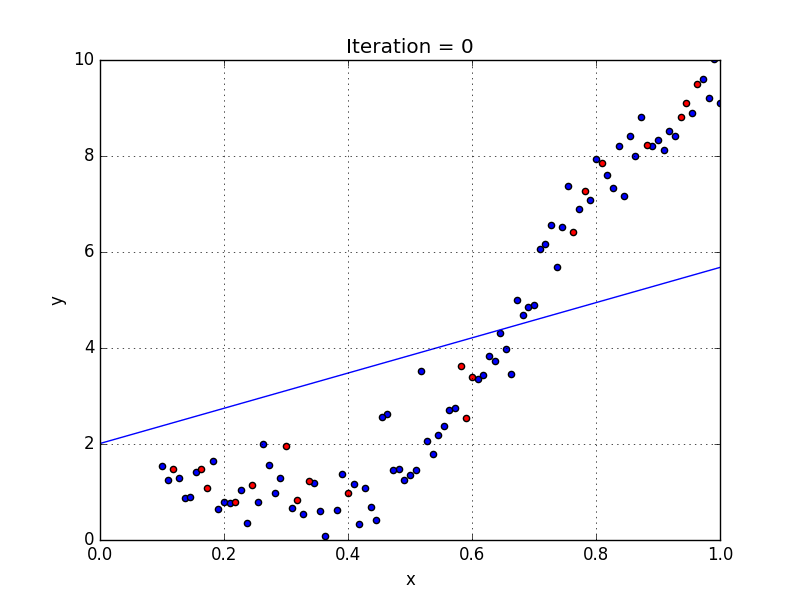## Build the Model

Remember that the final goal is always to discover and exploit the information that is hidden in the data. For this reason, since we have cleaned our data and checked that everything is in place we are going to build our first (super basic) machine learning model. The simplest option is the linear regression, you can’ t call yourself an acknowledged person in the Big Data world if you do not know what linear regression is. For this reason, I am going to provide a little bit of context here:

Linear regression attempts to model the relationship between two variables by fitting a linear equation to observed data. One variable is considered to be an explanatory variable, and the other is considered to be a dependent variable. For example, a modeler might want to relate the weights of individuals to their heights using a linear regression model.The equation of the line is: Y = a + bX, where X is the explanatory variable and Y is the dependent variable. The slope of the line is b, and a is the intercept (the value of y when x = 0).

``````import numpy as np
from sklearn.linear_model import LinearRegression
``````
``````##Load Dataset via Askdata

#IGNORE THIS
df1 = df.pop('alcohol') # remove column b and store it in df1
df['alcohol']=df1

X = df.iloc[:,:-1]
y = df.iloc[:,-1:]

``````
``````from sklearn.model_selection import train_test_split

X_train, X_test, y_train, y_test = train_test_split(X, y, test_size=0.33, random_state=42)
``````
``````reg = LinearRegression().fit(X_train, y_train)

reg.intercept_
reg.coef_

reg.score(X_test, y_test) #Returns R^2..not that bad
``````
``````0.7016255861966023
``````

Our mission is to make data accessible and useful to everyone.

AskData empowers people to interact with data in the most natural way: through natural language.

``````#  Please use the following credentials to login to the platform

``````
``````!pip install askdata
``````
``````from askdata import Askdata

agent_name = "titanic"
``````
``````Askdata Username: fosic56191@ppp998.com
``````
``````# Query askdata

askdata.get(query="sopravvissuti per classe", workspace = agent_name)
``````

Pclass , Survived from the dataset: titanic / Titanic_passengers

Pclass Survived
0 1 136
1 3 119
2 2 87
``````# or you can load the entire dataframe
df
``````

## Exercises

Given the titanic dataset

Variable Definition Key
survival Survival 0 = No, 1 = Yes
pclass Ticket class 1 = 1st, 2 = 2nd, 3 = 3rd
sex Sex
Age Age in years
sibsp # of siblings / spouses aboard the Titanic
parch # of parents / children aboard the Titanic
ticket Ticket number
fare Passenger fare
cabin Cabin number
embarked Port of Embarkation C = Cherbourg, Q = Queenstown, S = Southampton

b) Are there any Nan values? if Yes, please remove them since they are not relevant for the analysis.

c) print some useful information about the dataset. For example, The distribution of Survived w.r.t. Ticket class

d) Build a Logistic Regression model. (hint: clf = LogisticRegression(random_state=0).fit(X_train, y_train)

e) are you happy with the results?

``````from askdata import Askdata

agent_name = "titanic"
``````
``````Askdata Username: fosic56191@ppp998.com
``````
``````agent = askdata.agent(agent_name)
``````
``````[askdata_client.py:422 -  get_dataset_by_slug() ] - 2021-09-18 10:16:02,090 --> AUTH URL https://api.askdata.com/smartbot/agents/agentslug/titanic/datasetslug/titanic_passengers
``````
``````df.iloc[0,10]
df.isna().sum()
``````
``````''
``````
``````import matplotlib.pyplot as plt

### Bar plot

# create a figure and axis
# fig, ax = plt.subplots()
# # count the occurrence of each class
# data =df[df["Survived"]=="1"].groupby(["Pclass"]).count()["Survived"]
# # get x and y data
# points = data.index
# frequency = data.values
# # create bar chart
# ax.bar(points, frequency)
# # set title and labels
# ax.set_title('Titanic data')
# ax.set_xlabel('Survivors')
# ax.set_ylabel('Frequency')
# fig.show()
``````
``````df = agent.load_dataset("titanic_passengers")

## Handling Categorical Data in Python
## https://www.datacamp.com/community/tutorials/categorical-data Sezione Encoding Categorical Data

df1 = df[["Survived", "Pclass", "Age", "Sibsp", "Parch"]]

df1 = df1.replace(['N/A'], 28)

X = df1.iloc[:,:]
y = df1["Survived"]
del X["Survived"]

``````
``````[askdata_client.py:422 -  get_dataset_by_slug() ] - 2021-09-18 10:39:14,839 --> AUTH URL https://api.askdata.com/smartbot/agents/agentslug/titanic/datasetslug/titanic_passengers
``````
``````from sklearn.model_selection import train_test_split

X_train, X_test, y_train, y_test = train_test_split(X, y, test_size=0.33, random_state=42)
``````
``````from sklearn.linear_model import LogisticRegression

reg = LogisticRegression(random_state=0).fit(X_train, y_train)

reg.intercept_
reg.coef_

reg.score(X_test, y_test) #Returns R^2..not that bad
``````
``````0.7322033898305085
``````
``````reg.predict(X_test)[0:10]
``````
``````df.groupby(["Pclass","Survived"]).size()
``````
``````Pclass  Survived
1       0            80
1           136
2       0            97
1            87
3       0           372
1           119
dtype: int64
``````Tags: